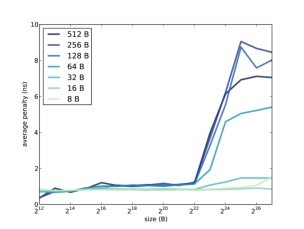# 7.4: Measuring cache performance

$$\newcommand{\vecs}{\overset { \rightharpoonup} {\mathbf{#1}} }$$ $$\newcommand{\vecd}{\overset{-\!-\!\rightharpoonup}{\vphantom{a}\smash {#1}}}$$$$\newcommand{\id}{\mathrm{id}}$$ $$\newcommand{\Span}{\mathrm{span}}$$ $$\newcommand{\kernel}{\mathrm{null}\,}$$ $$\newcommand{\range}{\mathrm{range}\,}$$ $$\newcommand{\RealPart}{\mathrm{Re}}$$ $$\newcommand{\ImaginaryPart}{\mathrm{Im}}$$ $$\newcommand{\Argument}{\mathrm{Arg}}$$ $$\newcommand{\norm}{\| #1 \|}$$ $$\newcommand{\inner}{\langle #1, #2 \rangle}$$ $$\newcommand{\Span}{\mathrm{span}}$$ $$\newcommand{\id}{\mathrm{id}}$$ $$\newcommand{\Span}{\mathrm{span}}$$ $$\newcommand{\kernel}{\mathrm{null}\,}$$ $$\newcommand{\range}{\mathrm{range}\,}$$ $$\newcommand{\RealPart}{\mathrm{Re}}$$ $$\newcommand{\ImaginaryPart}{\mathrm{Im}}$$ $$\newcommand{\Argument}{\mathrm{Arg}}$$ $$\newcommand{\norm}{\| #1 \|}$$ $$\newcommand{\inner}{\langle #1, #2 \rangle}$$ $$\newcommand{\Span}{\mathrm{span}}$$$$\newcommand{\AA}{\unicode[.8,0]{x212B}}$$

When I was a graduate student at U.C. Berkeley I was a teaching assistant for Computer Architecture with Brian Harvey. One of my favorite exercises involved a program that iterates through an array and measures the average time to read and write an element. By varying the size of the array, it is possible to infer the size of the cache, the block size, and some other attributes.

My modified version of this program is in the cache directory of the repository for this book (see Section 0.2).

The important part of the program is this loop:

    iters = 0;
do {
sec0 = get_seconds();

for (index = 0; index < limit; index += stride)
array[index] = array[index] + 1;

iters = iters + 1;
sec = sec + (get_seconds() - sec0);

} while (sec < 0.1);


The inner for loop traverses the array. limit determines how much of the array it traverses; stride determines how many elements it skips over. For example, if limit is 16 and stride is 4, the loop would access elements 0, 4, 8, and 12.

sec keeps track of the total CPU time used by the inner loop. The outer loop runs until sec exceeds 0.1 seconds, which is long enough that we can compute the average time with sufficient precision.

get_seconds uses the system call clock_gettime, converts to seconds, and returns the result as a double:

double get_seconds(){
struct timespec ts;
clock_gettime(CLOCK_PROCESS_CPUTIME_ID, &ts);
return ts.tv_sec + ts.tv_nsec / 1e9;
}Figure $$\PageIndex{1}$$: Average miss penalty as a function of array size and stride.

To isolate the time to access the elements of the array, the program runs a second loop that is almost identical except that the inner loop doesn’t touch the array; it always increments the same variable:

    iters2 = 0;
do {
sec0 = get_seconds();

for (index = 0; index < limit; index += stride)
temp = temp + index;

iters2 = iters2 + 1;
sec = sec - (get_seconds() - sec0);

} while (iters2 < iters);


The second loop runs the same number of iterations as the first. After each iteration, it subtracts the elapsed time from sec. When the loop completes, sec contains the total time for all array accesses, minus the total time it took to increment temp. This difference is the total miss penalty incurred by all accesses. Finally, we divide by the number of accesses to get the average miss penalty per access, in ns:

sec * 1e9 / iters / limit * stride


If you compile and run cache.c you should see output like this:

Size:    4096 Stride:       8 read+write: 0.8633 ns
Size:    4096 Stride:      16 read+write: 0.7023 ns
Size:    4096 Stride:      32 read+write: 0.7105 ns
Size:    4096 Stride:      64 read+write: 0.7058 ns


If you have Python and matplotlib installed, you can use graph_data.py to graph the results. Figure $$\PageIndex{1}$$ shows the results when I ran it on a Dell Optiplex 7010. Notice that the array size and stride are reported in bytes, not number of array elements.

Take a minute to consider this graph, and see what you can infer about the cache. Here are some things to think about:

• The program reads through the array many times, so it has plenty of temporal locality. If the entire array fits in cache, we expect the average miss penalty to be near 0.
• When the stride is 4 bytes, we read every element of the array, so the program has plenty of spatial locality. If the block size is big enough to contain 64 elements, for example, the hit rate would be 63/64, even if the array does not fit in cache.
• If the stride is equal to the block size (or greater), the spatial locality is effectively zero, because each time we read a block, we only access one element. In that case we expect to see the maximum miss penalty.

In summary, we expect good cache performance if the array is smaller than the cache size or if the stride is smaller than the block size. Performance only degrades if the array is bigger than the cache and the stride is large.

In Figure $$\PageIndex{1}$$, cache performance is good, for all strides, as long as the array is less than $$2^{22}$$ B. We can infer that the cache size is near 4 MiB; in fact, according to the specs, it is 3 MiB.

When the stride is 8, 16, or 32 B, cache performance is good. At 64 B it starts to degrade, and for larger strides the average miss penalty is about 9 ns. We can infer that the block size near 128 B.

Many processors use “multi-level caches” that include a small, fast cache and a bigger, slower cache. In this example, it looks like the miss penalty increases a little when the array size is bigger than $$2^{14}$$ B, so it’s possible that this processor also has a 16 KB cache with an access time less than 1 ns.

This page titled 7.4: Measuring cache performance is shared under a CC BY-NC license and was authored, remixed, and/or curated by Allen B. Downey (Green Tea Press) .